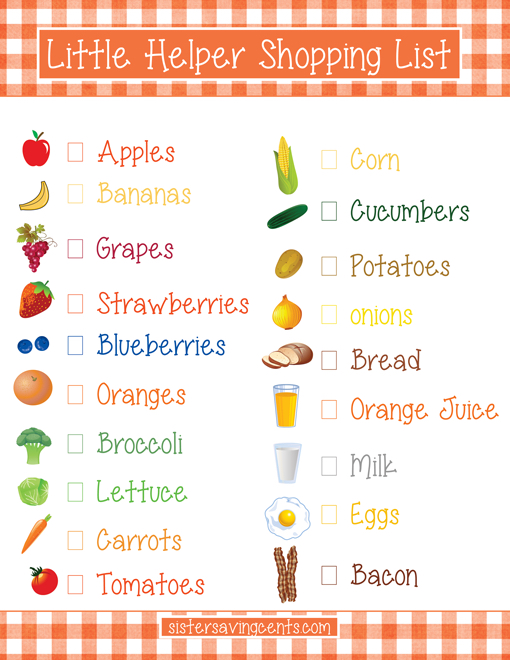In the previous chapter, you have learned to give names to expressions.

Until now, you have learned how to tell the computer to evaluate one expression. If you write several expression inside a box, the computer will display only the result of the last expression.

For instance, in this box:

``````(+ 3 4)
(* 7 8)
``````

The computer displays only the result of the evaluation of `(* 7 8)`.

In this chapter, you are going to learn how to tell the computer to display the result of several expressions. In computer language, it is called a `list` of expression.# A new friend: `list`

In order to tell the computer to evaluate several expressions, you need to use the operation named `list` and as the operands the expressions that you want to evaluate.

(You see, even when you tell the computer to evaluate several expressions, you write an expression. It is a bit confusing. Right?)

For that, we need to use the 3 steps of an expression:

1. parentheses
2. the name of the operation: `list`
3. the operands: the expressions that we want to evaluate

For instance, let’s tell the computer to display the evaluation of `(+ 3 4)` and `(* 7 8)`:

``````(list
(+ 3 4)
(* 7 8))
``````

Now, try to display the evaluation of `(+ 3 4)` and `(* 7 8)` and `(+ 9 8)`.

# list of lists

Now, how would you tell the computer to display several lists?

Simply like this:

``````(list
(list
(+ 3 4)
(* 7 8))

(list (+ 6 5)
(* 8 3)))

``````

And of course, inside a list, you can mix lists and numbers.

Like this:

``````(list
(list
(+ 3 4)
(* 7 8))

1 4 9)
``````

#Exercises

A. Display `2*3`, `2*4` and `2*5`.

``````()
``````

B. Display the numbers 1, 2, 3, 4 and 9

``````()
``````

C. Display the ages of your siblings (or friends)

``````()
``````

D. Display the age differences between you and your siblings (or friends)

``````()
``````

E. Display two lists:

1. the ages of your siblings
2. the odd numbers between 1 and 10
``````()
``````